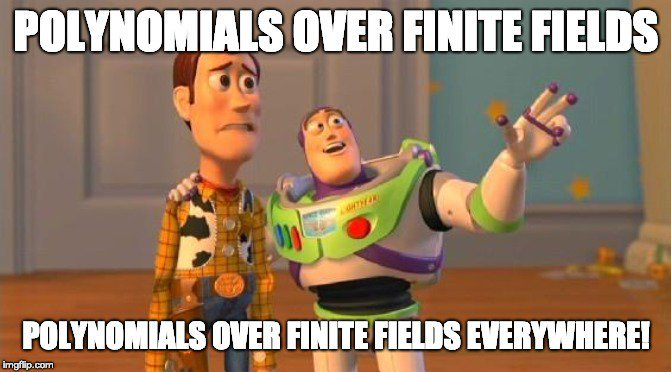# Digital signatures with oil and vinegar

“Unbalanced oil and vinegar” is a colorful name for a cryptographic signature method. This post will give a high-level description of the method and explain where the name comes from.

The RSA encryption algorithm depends on the fact that computers can easily multiply enormous numbers, but they cannot efficiently factor the product of two enormous primes. Whenever you have something that’s easy to do but hard to undo, you might be able to make an encryption algorithm out of it.

The unbalanced oil and vinegar (UOV) digital signature algorithm is analogous to RSA in that it also depends on the difficulty of factoring. But UOV is based on the difficulty of factoring the composition of a linear and nonlinear operator, not multiplying prime numbers. One advantage of UOV over RSA is that UOV is quantum-resistant. That is, if large quantum computers become practical, UOV signatures will remain hard to forge (or so it is currently believed) whereas RSA signatures would be easy to forge.

Solving large systems of multivariate polynomial equations over finite fields is hard, provably NP-hard, unless there’s some special structure that makes things easier. Several proposed post-quantum digital signature algorithms are based on this, such as the LUOV variant on UOV.

The idea behind UOV is to create systems of equations that have a special structure, with some “oil” variables and some “vinegar” variables, so named because they do not mix, or rather mix in a very simple, convenient way. This special structure is kept secret, and is obscured by composition with an invertible linear operator. This operator acts like a blender, thoroughly mixing the oil and vinegar. The term “unbalanced” refers to the fact that the scheme is more secure if you do not have equal numbers of “oil” and “vinegar” variables.Someone wanting to sign a file with the UOV algorithm knows the oil-and-vinegar structure and produces a vector that is mapped to a specified value, inverting the composition of the linear operator and the polynomials. They can do this because they know the factorization into this special structure. Someone wanting to verify a UOV signature only knows the (apparently unstructured) composition. They just see a large system of multivariate polynomial equations. They can stick a signature in and verify that the output is what it’s supposed to be, but they couldn’t produce a signature because they can’t invert the system. 

How large do these systems of polynomials need to be? On the order of a hundred equations and variables, though with more variables than polynomials. Not that large compared to linear systems, where one can efficiently solve systems with millions of equations and variables. And the polynomial are only quadratic. So in one sense the systems are small. But it takes several kilobytes  to describe such systems, which makes the public keys for UOV large relative to currently popular digital signature algorithms such as ECDSA. The signatures produced by UOV are small, but the public keys are large.

## Related posts

 The system is not invertible in the sense of being one-to-one because it’s underdetermined. By inverting the system we mean producing any input that maps to the desired output. This solution is not generally unique.

 Representing m quadratic polynomials in n variables over a field of size b bits requires bmn²/2 bits. So 80 quadratic polynomials in 120 variables over GF(28) would require 8 × 80 × 120²/2 = 4,608,000 bits = 576 kilobytes. The LUOV variation on UOV mentioned above reduces the key sizes quite a bit, but it still requires larger public keys than ECDSA.